Home Practice
For learners and parents For teachers and schools
Textbooks
Full catalogue
Pricing SupportLog in

We think you are located in United States. Is this correct?

# 6.7 Interpretation of graphs

## Worked example 22: Determining the equation of a parabola

Use the sketch below to determine the values of $$a$$ and $$q$$ for the parabola of the form $$y=a{x}^{2}+q$$.### Examine the sketch

From the sketch we see that the shape of the graph is a “frown”, therefore $$a<0$$. We also see that the graph has been shifted vertically upwards, therefore $$q>0$$.

### Determine $$q$$ using the $$y$$-intercept

The $$y$$-intercept is the point $$(0;1)$$.

\begin{align*} y& = a{x}^{2}+q \\ 1& = a{(0)}^{2}+q \\ \therefore q& = 1 \end{align*}

### Use the other given point to determine $$a$$

Substitute point $$(-1;0)$$ into the equation:

\begin{align*} y& = a{x}^{2}+q \\ 0& = a{(-1)}^{2}+1 \\ \therefore a& = -1 \end{align*}

$$a=-1$$ and $$q=1$$, so the equation of the parabola is $$y=-{x}^{2}+1$$.

## Worked example 23: Determining the equation of a hyperbola

Use the sketch below to determine the values of $$a$$ and $$q$$ for the hyperbola of the form $$y=\frac{a}{x}+q$$.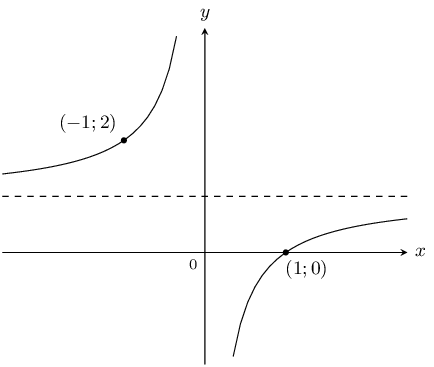### Examine the sketch

The two curves of the hyperbola lie in the second and fourth quadrant, therefore $$a<0$$. We also see that the graph has been shifted vertically upwards, therefore $$q>0$$.

### Substitute the given points into the equation and solve

Substitute the point $$(-1;2)$$:

\begin{align*} y& = \frac{a}{x}+q \\ 2& = \frac{a}{-1}+q \\ \therefore 2& = -a+q \end{align*}

Substitute the point $$(1;0)$$:

\begin{align*} y& = \frac{a}{x}+q \\ 0& = \frac{a}{1}+q \\ \therefore a& = -q \end{align*}

### Solve the equations simultaneously using substitution

\begin{align*} 2& = -a+q \\ & = q+q \\ & = 2q \\ \therefore q& = 1 \\ \therefore a& = -q \\ & = -1 \end{align*}

$$a=-1$$ and $$q=1$$, therefore the equation of the hyperbola is $$y=\dfrac{-1}{x}+1$$.

## Worked example 24: Interpreting graphs

The graphs of $$y=-{x}^{2}+4$$ and $$y=x-2$$ are given. Calculate the following:

1. coordinates of $$A$$, $$B$$, $$C$$, $$D$$

2. coordinates of $$E$$

3. distance $$CD$$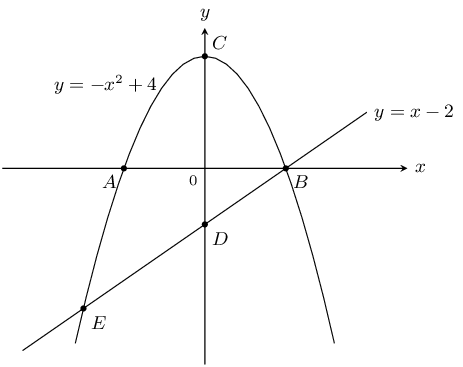### Calculate the intercepts

For the parabola, to calculate the $$y$$-intercept, let $$x=0$$:

\begin{align*} y& = -{x}^{2}+4 \\ & = -{0}^{2}+4 \\ & = 4 \end{align*}

This gives the point $$C(0;4)$$.

To calculate the $$x$$-intercept, let $$y=0$$:

\begin{align*} y& = -{x}^{2}+4 \\ 0& = -{x}^{2}+4 \\ {x}^{2}-4& = 0 \\ (x+2)(x-2)& = 0 \\ \therefore x& = ±2 \end{align*}

This gives the points $$A(-2;0)$$ and $$B(2;0)$$.

For the straight line, to calculate the $$y$$-intercept, let $$x=0$$:

\begin{align*} y& = x-2 \\ & = 0-2 \\ & = -2 \end{align*}

This gives the point $$D(0;-2)$$.

### Calculate the point of intersection $$E$$

At $$E$$ the two graphs intersect so we can equate the two expressions:

\begin{align*} x-2& = -{x}^{2}+4 \\ \therefore {x}^{2}+x-6& = 0 \\ \therefore (x-2)(x+3)& = 0 \\ \therefore x& = 2 \text{ or } -3 \end{align*}

At $$E$$, $$x=-3$$, therefore $$y=x-2=-3-2=-5$$. This gives the point $$E(-3;-5)$$.

### Calculate distance $$CD$$

\begin{align*} CD& = CO+OD \\ & = 4+2 \\ & = 6 \end{align*}

Distance $$CD$$ is $$\text{6}$$ units.

1. coordinates of $$A(-2;0)$$, $$B(2;0)$$, $$C(0;4)$$, $$D(0;-2)$$

2. coordinates of $$E(-3;-5)$$

3. distance $$CD = 6$$ units

## Worked example 25: Interpreting trigonometric graphs

Use the sketch to determine the equation of the trigonometric function $$f$$ of the form $$y=a f(\theta)+q$$.### Examine the sketch

From the sketch we see that the graph is a sine graph that has been shifted vertically upwards. The general form of the equation is $$y=a\sin\theta +q$$.

### Substitute the given points into equation and solve

At $$N$$, $$\theta =210°$$ and $$y=0$$:

\begin{align*} y& = a\sin\theta +q \\ 0& = a\sin 210°+q \\ & = a\left(-\frac{1}{2}\right)+q \\ \therefore q& = \frac{a}{2} \end{align*}

At $$M$$, $$\theta = 90°$$ and $$y=\frac{3}{2}$$:

\begin{align*} \frac{3}{2}& = a\sin90°+q \\ & = a+q \end{align*}

### Solve the equations simultaneously using substitution

\begin{align*} \frac{3}{2}& = a+q \\ & = a+\frac{a}{2} \\ 3& = 2a+a \\ 3a& = 3 \\ \therefore a& = 1 \\ \therefore q& = \frac{a}{2} \\ & = \frac{1}{2} \end{align*}

$$y=\sin\theta +\frac{1}{2}$$
Textbook Exercise 6.7

Plot the following functions on the same set of axes and clearly label all the points at which the functions intersect.

$$y = x^2 +1$$ and $$y = 3^x$$

The $$y$$-intercept for each graph is:

\begin{aligned} 0^2 + 1 & = 1 \\ 3^0 & = 1 \end{aligned}

This is also the only point of intersection.

For both graphs there is no $$x$$-intercept.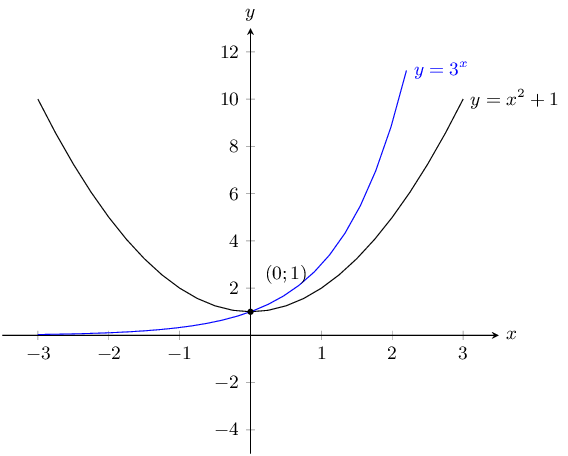$$y = x$$ and $$y = \dfrac{2}{x}$$

$$y = x$$ is a basic straight line graph. For $$y =\frac{2}{x}$$ there is no $$y$$-intercept and no $$x$$-intercept. We note that this is a hyperbolic graph that has been stretched by 2 units.

The points of intersection are:

\begin{align*} x &= \frac{2}{x} \\ x^2 &= 2 \\ x^2 - 2 & = 0\\ (x - \sqrt{2})(x + \sqrt{2}) & = 0\\ x = \sqrt{2} &\text{ or } x = -\sqrt{2} \\ y = \sqrt{2} &\text{ or } y = -\sqrt{2} \end{align*}

The graphs intersect at $$\left(\sqrt{2};\sqrt{2}\right)$$ and $$\left(-\sqrt{2};-\sqrt{2}\right)$$.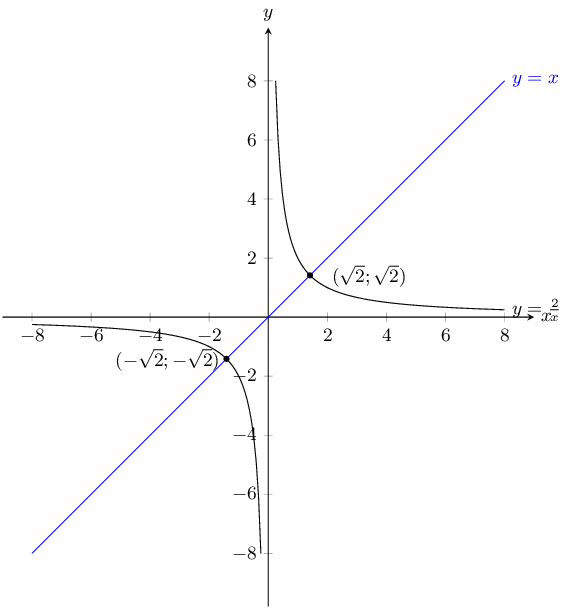$$y = x^2 +3$$ and $$y = 6$$

$$y = 6$$ is a horizontal line through $$(0;6)$$. For $$y =x^2 + 3$$ the $$y$$-intercept is $$(0;3)$$ and there are no $$x$$-intercepts. From the value of $$q$$ we see that this is a basic parabola that has been shifted upwards by 3 units.

The points of intersection are:

\begin{align*} x^2 + 3 &= 6 \\ x^2 - 3 &= 0 \\ (x - \sqrt{3})(x + \sqrt{3}) & = 0\\ x = \sqrt{3} &\text{ or } x = -\sqrt{3} \\ y = 6 \end{align*}

The graphs intersect at $$\left(\sqrt{3};6\right)$$ and $$\left(-\sqrt{3};-6\right)$$.$$y = -x^2$$ and $$y = \dfrac{8}{x}$$

$$y = -x^{2}$$ is a parabola that has been reflected about the $$x$$-axis. For $$y =\frac{8}{x}$$ there is no $$y$$-intercept and there is no $$x$$-intercepts. From the value of $$a$$ we see that this is a basic hyperbola that has been stretched by 8 units.

The points of intersection are:

\begin{align*} -x^2 &= \frac{8}{x} \\ x^3 &= - 8 \\ x & = -2\\ y &= \frac{8}{-2} \\ y &= -4 \end{align*}

The graphs intersect at $$\left(-2;-4\right)$$.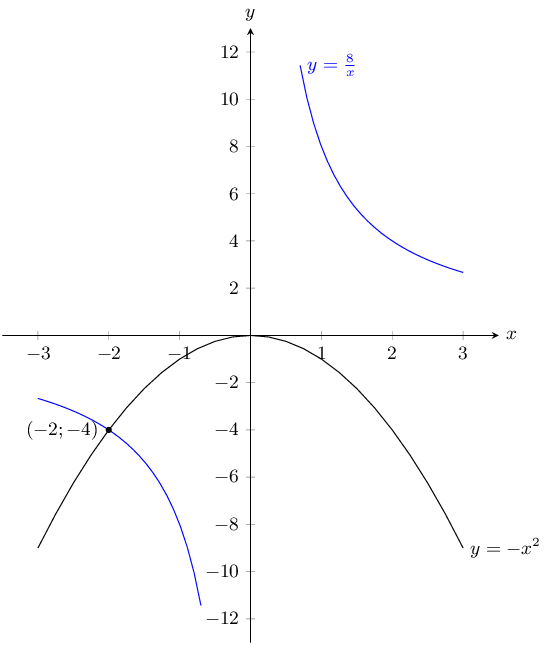Determine the equations for the graphs given below.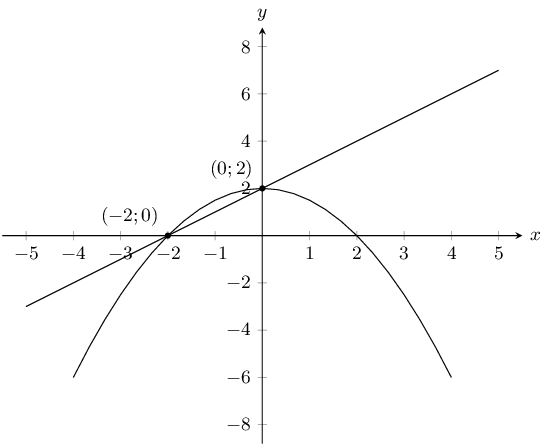For the straight line graph we have the $$x$$ and $$y$$-intercepts. The $$y$$-intercept gives $$c = 2$$. Now we can calculate the gradient of the straight line graph:

\begin{align*} y &= mx + 2 \\ m &= \frac{2 - 0}{0 - (-2)} \\ &= 1 \end{align*}

Therefore the equation of the straight line graph is $$y = x + 2$$.

For the parabola we also have the $$x$$ and $$y$$-intercepts. The $$y$$-intercept gives $$q = 2$$. Now we can calculate $$a$$:

\begin{align*} y &= ax^2 + 2 \\ 0 &= a(-2)^2 + 2 \\ -2 &= 4a \\ a &= -\frac{1}{2} \end{align*}

Therefore the equation of the parabola is $$y = -\frac{1}{2}x^2 + 2$$.

The equations for the two graphs are $$y = x + 2$$ and $$y = -\frac{1}{2}x^2 + 2$$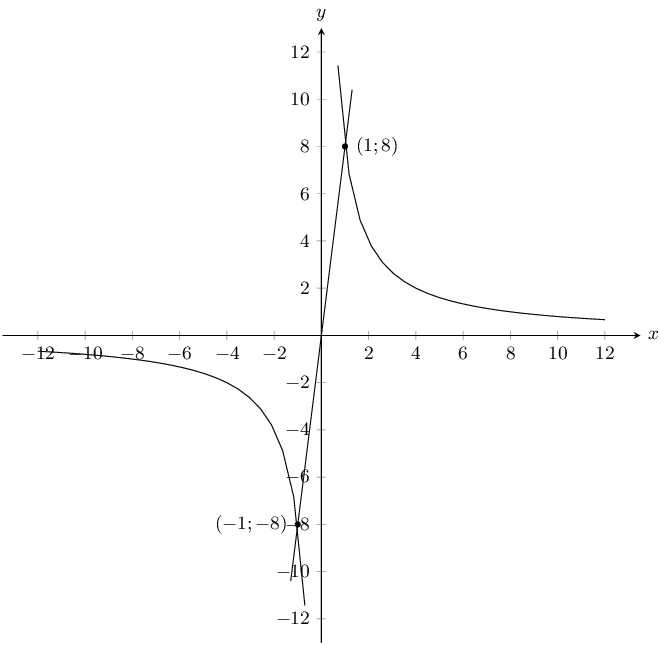For the straight line graph we notice that it passes through $$(0;0)$$ and so $$c = 0$$.

We have two points on the straight line graph and so we can calculate the gradient, $$m$$:

\begin{align*} y &= mx + 0 \\ m &= \frac{8 - (-8)}{1 - (- 1)} \\ m &= 8 \end{align*}

The equation of the straight line graph is $$y = 8x$$.

For the hyperbola we note that the graph is not shifted either upwards or downwards. Therefore $$q = 0$$. Now we can calculate $$a$$:

\begin{align*} y &= \frac{a}{x} \\ 8 &= \frac{a}{1} \\ a &= 8 \end{align*}

Therefore the equation of the hyperbola is $$y = \frac{8}{x}$$.

The equations for the two graphs are $$y = 8x$$ and $$y = \frac{8}{x}$$.

The range of $$y = 2 \sin{\theta} + 1$$ is:

1. $$1 \le \theta \le 2$$
2. $$-2 \le \theta \le 2$$
3. $$-1 \le \theta \le 3$$
4. $$-2 \le \theta \le 3$$

3

The range of $$y = 2 \cos{\theta} - 4$$ is:

1. $$-6 \le \theta \le 2$$
2. $$-4 \le \theta \le -2$$
3. $$-6 \le \theta \le 1$$
4. $$-6 \le \theta \le -2$$

4

The y-intercept of $$2^x + 1$$ is:

1. $$3$$
2. $$1$$
3. $$2$$
4. $$0$$

3

Which of the following passes through $$(1;7)$$?

1. $$y = \frac{7}{x}$$
2. $$y = 2x + 3$$
3. $$y = \frac{4}{x}$$
4. $$y = x^2 + 1$$

1